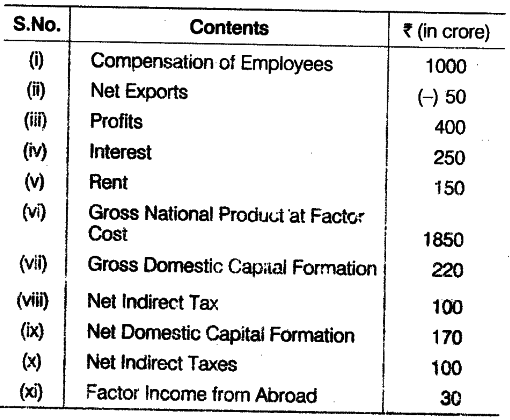# Calculate Gross Domestic Product at Market Price

Calculate (a) Gross Domestic Product at Market Price and (b) Factor income to abroad from the following data:(a) \$ND{{P}{FC}}\$ = Compensation of Employees + Rent+ Interest + Profits = 1000 +150+250 + 400 =
Rs. 1800 crore
\$GD{{P}
{MP}}\$ =
\$ND{{P}{FC}}\$ + Net Indirect Taxes + Depreciation = 1800 +100 + (220 -170) = Rs. 1950 crore
(b) \$GN{{P}
{FC}}\$ =
\$GD{{P}_{MP}}\$ - Net Indirect Taxes